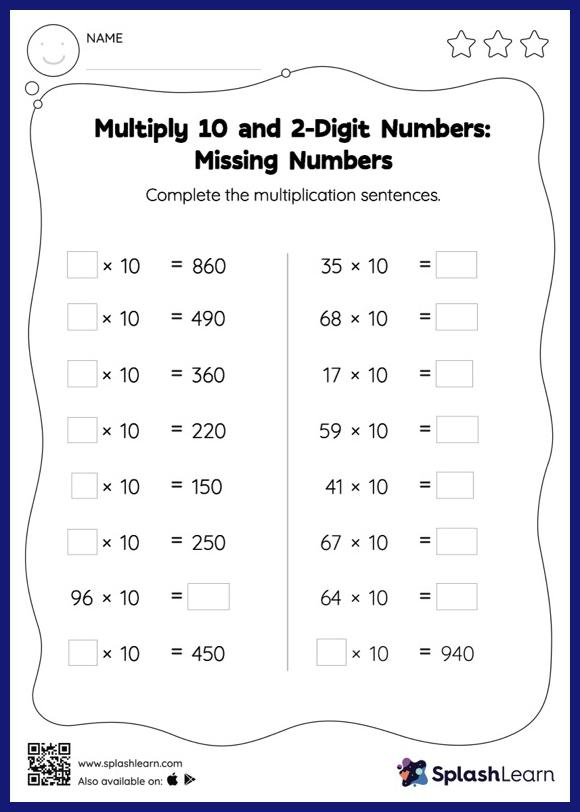# Multiply 10 and 2-Digit Numbers: Missing Numbers Worksheet

Home > Multiply 10 and 2-Digit Numbers: Missing NumbersThis multiply 10 and 2-digit numbers worksheet has a set of problems curated to ensure that your child becomes more proficient in multiplication. Students love it when they get to multiply numbers by 10, 100, or their multiples. They use the pattern of zeros to find the product. In multiply 10 and 2-digit numbers worksheet, they use the same concept to find the missing number in multiplication sentences.